# Mixed to improper

Change the given mixed numbers to improper fraction: five-and-four-over-nine (5 4/9)

x =  5.4444

### Step-by-step explanation: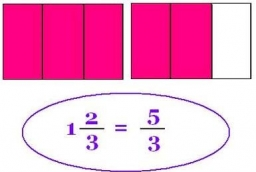Did you find an error or inaccuracy? Feel free to write us. Thank you!Tips to related online calculators
Need help to calculate sum, simplify or multiply fractions? Try our fraction calculator.

## Related math problems and questions:

• To improper fractionChange mixed number to improper fraction a) 1 2/15 b) -2 15/17
• Mixed2improperWrite the mixed number as an improper fraction. 166 2/3
• Fractions and mixed numerals(a) Convert the following mixed numbers to improper fractions. i. 3 5/8 ii. 7 7/6 (b) Convert the following improper fraction to a mixed number. i. 13/4 ii. 78/5 (c) Simplify these fractions to their lowest terms. i. 36/42 ii. 27/45 2. evaluate the follow
• Mixed numbersRewrite mixed numbers, so the fractions have the same denominator: 5 1/5 - 2 2/3
• 6/24 change6/24 change to a fraction in lowest terms
• Divide 13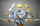Divide. Simplify your answer and write as an improper fraction or whole number. 14÷8/3
• Evaluate 17Evaluate 2x+6y when x=- 4/5 and y=1/3. Write your answer as a fraction or mixed number in simplest form.
• A turtle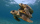A turtle is 20 5/6 inches below the surface of a pond. It dives to a depth of 32 1/4 inches. What is the change in the turtle’s position? Write your answer as a mixed number.
• Oil change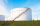A container holds 1/4 gallon of oil. Jack is changing the oil in his vehicles and needs 11 containers. How many gallons of oil does Jack need?
• Change the numbers in the ratio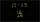Change the numbers 29, 38, and 43 in a 3: 4 ratio.
• Product of two fractionsProduct of two fractions is 9 3/5 . If one of the fraction is 9 3/7. Find the other fraction.
• FractionFind for what x fraction (-4x -6)/(x) equals:
• Divide 11Divide the product of 4 and 5/8 by 1 1/2. Write your answer as a mixed number.Add two mixed fractions: 2 4/6 + 1 3/6Simplify mixed numerals expression: 8 1/4- 3 2/5 - (2 1/3 - 1/4) Show your solution.Add this two mixed numbers: 1 5/6 + 2 2/11=Estimate the answer by rounding each fraction to the nearest whole number and then subtracting. 9/15 - 2 7/8